Practice the AP 10th Class Maths Bits with Answers Chapter 2 Sets on a regular basis so that you can attempt exams with utmost confidence.

## AP State Syllabus 10th Class Maths Bits 2nd Lesson Sets with Answers

Question 1.
From the Venn diagram, A ∪ B = …………….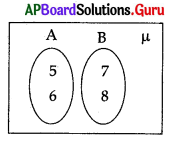A) {5, 6}
B) {5, 6, 7, 8}
C) Φ
D) {7,8}
B) {5, 6, 7, 8}

Question 2.
If A ⊆ B, then which one of following is false ?
A) A – B = A
B) B – A ≠ Φ
C) A ∪ B = B
D) A ∩ B = A
B) B – A ≠ ΦQuestion 3.
If A ⊂ B, then A ∩ B = ……………….
A) A
B) B
C) Φ
D) µ
A) A

Question 4.
The number of subsets of a set is 16, then the set has …………………. elements.
A) 1
B) 2
C) 3
D) 4
D) 4

Question 5.
{x: x is a prime number and a divisor of 6} =…………………..
A) {1, 2, 3, 6}
B) {1, 2, 3}
C) {2, 3}
D) {2, 3, 6}
C) {2, 3}

Question 6.
Number of subsets of the set A = {1, 2, 3, 4} is ………………
A) 4
B) 8
C) 12
D) 16
D) 16

Question 7.
If A = {x : x is a letter in the word HEADMASTER}; then its Roster form is ……………………
A) A = {h, e, a, d, m, a, s, t, e, r}
B) A = {h, e, a, d, m, s, t, r}
C) A = {h, e, a, d, m, s, t, e, r}
D) A = {h, e, a, d, m, a, s, t, r}
B) A = {h, e, a, d, m, s, t, r}

Question 8.
The following Venn diagram indicates ………………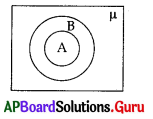A) A ⊂ B
B) B ⊂ A
C) A, B are disjoint sets
D) µ ⊂ B
A) A ⊂ B

Question 9.
If n(A) = 12 and n(A ∩ B) = 5, then find n(A – B) = …………………
A) 4
B) 7
C) 17
D) 0
B) 7Question 10.
If A ⊂ B then (A ∪ B) – A = ……………………..
A) B
B) Φ
C) A
D) B – A
D) B – A

Question 11.
If A ⊂ B and B ⊂ C then ……………………
A) A ⊂ C
B) C ⊂ A
C) A = C
D) B = A
A) A ⊂ C

Question 12.
If A = {x/x∈N and 1 < x < 6}, then n(A) =
A) 6
B) 5
C) 4
D) 2
C) 4

Question 13.
S = $$\left\{3, \pi, \sqrt{2},-5,3+\sqrt{7}, \frac{2}{7}\right\}$$. Which of the following is a sub-set of ‘S’ containing all irrational numbers ?
A) $$\left\{3, \pi, \frac{2}{7},-5,3+\sqrt{7}\right\}$$
B) $$\{3+\sqrt{7}, \sqrt{2}, \pi\}$$
C) $$\{3, \pi, \sqrt{2}\}$$
D) $$\left\{3,-5, \frac{2}{7}\right\}$$
B) $$\{3+\sqrt{7}, \sqrt{2}, \pi\}$$

Question 14.
The symbol for an empty set is
A) : :
B) Φ
C) :Φ:
D) A or B
B) Φ

Question 15.
If A ∩ B = Φ, then the sets A, B are
A) equal sets
B) sub sets
C) disjoint sets
D) equivalent sets
C) disjoint sets

Question 16.
If A = {1, 2, 3, 4, 5} and B = {4, 5, 6, 7], then A – B =
A) {4, 5}
B) {6,7}
C) {1, 2, 3}
D) {1, 2, 3, 4, 5, 6, 7}
C) {1, 2, 3}Question 17.
Among the following, a null set is (where N is the set of natural numbers)
A) {x : x < 5 and x ∈ N)
B) {x : x2 = 4, x ∈ N}
C) {x : x2 + 1 = 0 x ∈ N}
D) {x : x is even prime}
C) {x : x2 + 1 = 0 x ∈ N}

Question 18.
If A ⊂ B, then A – B =
A) B
B) Φ
C) A
D) B – A
B) Φ

Question 19.
If A = {1, 2, 3, 4, 5, 6}, B = {4, 5, 6, 7, 8}, C = {4, 5, 6}, then A ∩ B =
A) A
B) B
C) C
D) None
C) C

Question 20.
If A and B are subsets of a universal set μ, then A ∩ Bc =
A) A – B
B) A∪B
C) Φ
D) μ
A) A – B

Question 21.
If n(A) = 15, n(B) = 10, n(A ∩ B) = 5, then n(A ∪ B) =
A) 5
B) 15
C) 20
D) 25
C) 20

Question 22.
If A ⊂ B and B ⊂ C, then (A ∩ B) ∪ C =
A) Φ
B) A
C) B
D) C
D) C

Question 23.
If A = {x/x∈N, 3 ≤ x ≤ 6}, then A =
A) {3, 4, 5}
B) {3, 4, 5, 6}
C) {4, 5}
D) {4, 5, 6}
B) {3, 4, 5, 6}Question 24.
If A = [x/x is an even natural number less than 12} and B = {x/x is a prime number less than 12}, then A∩B=
A) {2, 4, 6, 8, 10}
B) {2, 3, 5, 7}
C) Φ
D) {2}
D) {2}

Question 25.
The set builder form of the set $$\left\{1, \frac{2}{7}, \frac{3}{17}, \frac{4}{31}, \frac{5}{49}\right\}$$ is …………………
A) $$\left\{\frac{x}{x^{2}-1} / x \in N ; x \leq 5\right\}$$
B) $$\left\{\frac{x}{2 x^{2}-1} / x \in N ; x<5\right\}$$
C) $$\left\{\frac{x}{2 x^{2}-1} / x \in N ; x \leq 5\right\}$$
D) $$\left\{\frac{x}{x^{3}-1} / x \in N ; x \leq 5\right\}$$
C) $$\left\{\frac{x}{2 x^{2}-1} / x \in N ; x \leq 5\right\}$$

Question 26.
If A and B are two sets then (A – B) ∩ (B – A) =
A) A
B) B
C) A Δ B
D) Φ
D) Φ

Question 27.
The following Venn-diagram design represented by C equals to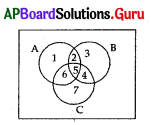A) {1, 2, 5, 6}
B) {6, 5}
C) {4, 5, 6, 7}
D) {2, 5, 6}
C) {4, 5, 6, 7}

Question 28.
The shaded area in the figure is
IM4
A) A ∩ (B ∪ C)
B) A ∩ (B ∩ C)
C) A ∪ (B ∩ C)
D) A ∪ (B ∪ C)
A) A ∩ (B ∪ C)

Question 29.
If A is a subset of B, then A – B = ………………..
A) A
B) B
C) B – A
D) Φ
D) Φ

Question 30.
Set builder form of {1, 3, 5, 7…. 19}
A) {x/x is odd number and x ≤ 19}
B) {2x/x ∈ N,x ≤ 1}
C) {x + 2/x ∈ N}
D) {x/x is even number and x > 19}
A) {x/x is odd number and x ≤ 19}

Question 31.
Which one of the following sets are equal?
A) A = {1, 0}; B = {a, b}
B) C = {0, a}; D = {b, 0}
C) E = {4, 8, 12}; F = {8, 12, 4}
D) G = {2, 5}; H = {5, 2, 1}
C) E = {4, 8, 12}; F = {8, 12, 4}Question 32.
The symbol for “implies” is ……………….
A) ⇒
B) ⇔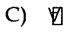D) ∃
A) ⇒

Question 33.
Two sets A and B are said to be disjoint, if ………………….
A) A ∪ B = Φ
B) A – B = Φ
C) B – A = Φ
D) A ∩ B = Φ
D) A ∩ B = Φ

Question 34.
In Venn diagram given below: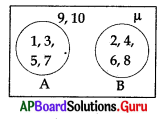A) A ∪ B = Φ
B) A ∪ B = μ
C) A ∩ B = μ
D) A ∩ B = Φ
D) A ∩ B = Φ

Question 35.
Set A = {F, L, W, O} which of the following is not a set-builder form for set A ?
A) {x : x is a letter from the word ‘FOLLOW’}
B) {x:x is a letter from the word’FLOW’}
C) {x: x is a letter from the word ‘WOLF’}
D) {x: x is a letter from the word ‘SLOW’}
D) {x: x is a letter from the word ‘SLOW’}

Question 36.
If A = {1, 2, 2, 1, 3, 4, 3, 4}, then n(A) =
A) 8
B) 0
C) 4
D) 20
C) 4

Question 37.
A = Set of divisors of 3, B = Set of divisors of 6, C = Set of divisors of 2, then
A) A ⊆ C
B) C ⊆ B
C) B ⊆ A
D) A ⊆ B
C) B ⊆ AQuestion 38.
If A = {1, 2, 3} , then the number of elements in p(A) is
A) 6
B) 3
C) 8
D) 0
C) 8

Question 39.
If n(A) = 5, then n[p(A)] =
A) 0
B) 5
C) 32
D) 25
C) 32

Question 40.
If A is any set such that n[p(A)] = 64, then n(A) =
A) 6
B) 16
C) 32
D) 8
A) 6

Question 41.
If p(A) contains 128 elements, then the number of elements in A is
A) 32
B) 7
C) 16
D) 64
B) 7

Question 42.
If A = {1, 2, 3}, B = {2, 3, 4}, then A ∪ B =
A) {4}
B) {2, 3}
C) {1, 2, 3, 4}
D) {1}
C) {1, 2, 3, 4}

Question 43.
If A = {1, 2, 3}, B = {2, 3, 4}, then A ∩ B =
A) {1, 2, 3, 4}
B) {4}
C) {1}
D) {2, 3}
D) {2, 3}

Question 44.
If A = {1, 2, 3, 4}, B = {2, 4, 6, 8}, C = {3, 4, 5, 8}, then A∩B∩C =
A) {4}
B) {2, 4}
C) μ
D) Φ
A) {4}Question 45.
If A = {x : x is a factor of 15}, B = |x : x is a factor of 18}, then A∩B =
A) {1, 3}
B) {1, 3, 5, 15}
C) {1, 2, 3, 6, 9, 18}
D) {5, 15}
A) {1, 3}

Question 46.
If A = {1, 2, 3}, B = {2, 3, 4}, then A – B =
A) {4}
B) {1}
C) {2, 3}
D) {1, 2, 3, 4}
B) {1}

Question 47.
If A = {1,2, 3}, B = {2, 3,4}, then B – A =
A) {1, 2, 3, 4}
B) {2, 3}
C) {4}
D) {1}
C) {4}

Question 48.
If A = {1, 2, 3, 4}, B = {2, 4, 6, 8}, C = {3, 4, 5, 8}, then A – (B ∪ C) =
A) {1}
B) μ
C) A
D) Φ
A) {1}

Question 49.
If A = {3, 5, 7, 9, 4}, B = {1, 3, 5}, C = {2, 4, 6, 7} then A – (B ∩ C) =
A) C
B) A
C) B
D) Φ
B) A

Question 50.
If A = {3, 5, 7, 9}, B = {1, 3, 5}, μ = {1, 2, 3, 4, 5 , 7, 9} then (A ∪ B)’ =
A) {2, 4, 6, 8}
B) {1, 2, 4, 7, 9}
C) Φ
D) {2, 4}
D) {2, 4}

Question 51.
If μ = {1, 2, 3, 4, 5, 6, 7, 8} A = {1, 2, 4, 5, 6} then A’ =
A) {3, 7}
B) {3, 7, 8}
C) {7, 8}
D) {5, 6, 8}
B) {3, 7, 8}Question 52.
If n(A) = 20, n(B) = 44, n(A ∪ B) = 51, then n(A ∩ B) =
A) 24
B) 22
C) 13
D) 39
C) 13

Question 53.
If n(A) = 20, n(B) = 44 , n(A ∩ B) = 13 then n(A ∪ B) =
A) 24
B) 51
C) 22
D) 59
B) 51

Question 54.
If n(A) = 35, n(B) = 25, n(A ∪ B) = 45, then n(A ∩ B) =
A) 20
B) 25
C) 10
D) 15
D) 15

Question 55.
If n(A) = 37, n(B) = x, n(A ∪ B) = 52, n(A ∩ B) = 8, then x =
A) 23
B) 15
C) 29
D) 44
A) 23

Question 56.
If A ⊆ B , n(A) = 21 , n(B) = 41, then n(A ∪ B)=
A) 62
B) 41
C) 21
D) 20
B) 41

Question 57.
If A ⊆ B, n(A) = 25, n(B) = 35, then n(A ∩ B) =
A) 60
B) 25
C) 10
D) 35
D) 35

Question 58.
If n(A) = 12, n(B) = 16, n(C) = 21,
n(A ∩ B) = 5, n(A ∩ C) = 8,
n(B ∩ C) = 12, n(A ∩ B ∩ C) = 3, then n(A ∪ B ∪ C) =
A) 27
B) 34
C) 31
D) 59
A) 27Question 59.
If A = {prime numbers} , B = {even numbers}, then n(A ∩ B) =
A) Φ
B) 2
C) 1
D) 0
C) 1

Question 60.
90 students take Mathematics, 72 take Science in a class of 120 students. If 10 take neither Mathematics nor Science then the number of students take both the subjects is
A) 110
B) 100
C) 52
D) 162
C) 52

Question 61.
Let μ = all triangles , A = all isosceles triangles, B = all equilateral triangles, C = all right angle triangles, then the angles belongs to the set A∩C is
A) 90°, 30°, 60°
B) 90°, 45°, 45°
C) 60°, 60°, 60°
D) 50°, 60°, 70°
B) 90°, 45°, 45°

Question 62.
In a school of 214 students 134 takes tea, 134 takes coffee. Then how many takes only tea or coffee
A) 170
B) 180
C) 54
D) 160
D) 160

Question 63.
Which of the following is an empty set?
A) {0}
B) The set of all even prime numbers
C) The set of all natural numbers less than 1
D) {Φ}
C) The set of all natural numbers less than 1

Question 64.
If A = {1, 2, 3, 4}, B = {x, y, z, w} then A and B are
A) Empty sets
B) Equal sets
G) Complementary sets
D) Equivalent sets
D) Equivalent sets

Question 65.
Which of the following is not a set ?
A) The collection of all intelligent boys in a class
B) The collection of all boys of age greater than 10 years
C) The collection of all boys of height less than 100 cms
D) The collection of all girls in a class
A) The collection of all intelligent boys in a class

Question 66.
Which of the following is an infinite set?
A) The set of all natural numbers from 1 to 1 crore
B) The set of all multiples of 5
C) The set of all natural numbers less than 100
D) The set of all divisors of 240
B) The set of all multiples of 5Question 67.
Which of the following is not a subset of {2, 3, 5, 7} ?
A) {3, 5}
B) {2, 3, 5}
C) {3, 5, 7}
D){1, 3, 5}
D){1, 3, 5}

Question 68.
If n(A) = 8, n(B) = 5, then
A) A ⊆ B
B) B ⊆ A
C) There exists three elements in A which are not in B
D) A ⊇ B
D) A ⊇ B

Question 69.
Which of the following sets has only one subset ?
A) {0, 1}
B) {0}
C) {1}
D) { }
A) {0, 1}

Question 70.
Two sets A and B are disjoint sets, if and only if
A) A ∩ B = B
B) A – B = A
C) A – B = Φ
D) A ∪ B = Φ
B) A – B = A

Question 71.
If A and B are disjoint, then A ∩ (B ∩ C) =
A) μ
B) A
C) Φ
D) B
C) Φ

Question 72.
If A ⊂ B, then A ∪ B=
A) A
B) μ
C) Φ
D) B
D) B

Question 73.
If A ⊂ B, then A ∩ B =
A) Φ
B) A
C) μ
D) B
B) AQuestion 74.
A is a set . Which of the following is false? ‘
A) A ∩ A = A
B) A ∪ A = A
C) A ∩ Φ = A
D) A ∪ Φ = A
C) A ∩ Φ = A

Question 75.
If A = {Rectangles}, B = {Rhombuses}, then A ∪ B =
A) {Rhombuses}
B) {Rectangles}
C) {Squares}
D) {Triangles}
B) {Rectangles}

Question 76.
If A = {Rhombuses}, B = {Rectangles}, then A ∩ B =
A) {Parallelograms}
B) {Rhombuses}
C) {Squares}
D) {Rectangles}
C) {Squares}

Question 77.
If A “{Equilateral triangles}, B = {Right angled triangles} and C = {Isosceles triangles}, then
A) A ∩ C = Φ
B) A ∩ B = Φ
C) B ∩ C = Φ
D) None
D) None

Question 78.
If l,s are two straight lines and l ∩ s = Φ then l and s are
A) parallel
B) coincide
C) perpendicular
D) similar
A) parallel

Question 79.
Which of the following is correct? :
A) (A ∪ B)’ = A’ ∩ B’
B) (A ∪ B)’=A ∪ B
C) (A ∪ B)’ = A’ ∩ B
D) (A ∪ B)’ = A ∪ B’
A) (A ∪ B)’ = A’ ∩ B’

Question 80.
Which of the following is false ?
A) A ∩ A’= Φ
B) A ∩ Φ = Φ
C) A ∩ μ = μ
D) A ∩ A = A
C) A ∩ μ = μQuestion 81.
If A ⊆ B, then
A) B1 ⊆ A1
B) B ⊆ A1
C) A ⊆ B1
D) A1 ⊆ B1
A) B1 ⊆ A1

Question 82.
If A ∪ B = Φ then
A) A = Φ or B = Φ
B) A = Φ
C) A = Φ and B = Φ
D) B = Φ
C) A = Φ and B = Φ

Question 83.
If (A – B) ⊆ (B – A) then
A) B ⊆ A
B) A ∪ B = C
C) A – B = B – A
D) A ⊆ B
D) A ⊆ B

Question 84.
If A, B are two sets then which of the following is false ?
A) A – (A – B) = A ∩ B
B) A – B’ = A ∩ B
C) A ⊂ B ⇒ B’ ⊆ A’
D) A – B = B- A
D) A – B = B- A

Question 85.
If n(A) = 8, n(B) = 5 then minimum number of elements in A ∪ B is …………….
A) 9
B) 8
C) 13
D) 5
B) 8

Question 86.
Let p be the set of all prime numbers between 0 and 100 then cordinality of p is ………………..
A) 50
B) 101
C) 25
D) 24
C) 25

Question 87.
If A = {2, 3, 4, 5}, B ={3, 4, 5}, C – {2, 5} then which of the following is null set?
A) B ∩ C
B) A – B
C) (B ∩ C) – A
D) B – C
C) (B ∩ C) – AQuestion 88.
An object of a set is called
A) element
B) subject
C) number
D) alphabet
A) element

Question 89.
{x: x ∈ N, x lies between 5 and 12} =
A) {5, 6, ……………. 12}
B) {6, 8, 10}
C) {5, 12}
D) {6, 7, 8, 9, 10, 11}
D) {6, 7, 8, 9, 10, 11}

Question 90.
Empty set is
A) Not exist
B) Unique
C) Infinite
D) Unique if it exists
B) Unique

Question 91.
The number of elements in the empty set is
A) 1
B) 0
C) ∞
D) Φ
B) 0

Question 92.
If n(A) represents the number of elements in the set A, the number of proper subsets of A are
A) 2 × n
B) 2n – 1
C) n × A
D) 2n
B) 2n – 1

Question 93.
A ∪ B =
A) {x : x ∉ A or x ∈ B}
B) {x : x ∈ A or x ∈ B}
C) {x : x ∈ A, x ∈ B}
D) {x : x ∈ A or x ∉ B}
B) {x : x ∈ A or x ∈ B}

Question 94.
A ∩ B =
A) {x : x ∈ A or x ∈ B}
B) {x : x ∉ A or x ∈ B}
C) {x : x ∈ A, x ∈ B}
D) {x : x ∈ A or x ∉ B}
C) {x : x ∈ A, x ∈ B}Question 95.
If A and B are disjoint, then A ∩ B
A) μ
B) A
C) B
D) Φ
D) Φ

Question 96.
A ∩ Φ =
A) Φ
B) A
C) Ac
D) μ
A) Φ

Question 97.
A ∪ Φ =
A) μ
B) A
C) Ac
D) Φ
B) A

Question 98.
Identity of union of sets is
A) 0
B) μ
C) A
D) Φ
D) Φ

Question 99.
Identity of intersection of sets is
A) μ
B) A
C) 0
D) Φ
A) μ

Question 100.
If x ∉ A ∪ B, then
A) x ∉ A, x ∉ B
B) x ∈ A ,x ∉ B
C) x ∉ A or x ∉ B
D) x ∈ A, x ∈ B
C) x ∉ A or x ∉ B

Question 101.
A ∪ ( B ∩ C) =
A) (A ∪ B) ∪ (A ∩ C)
B) A ∩ (B ∪ C)
C) (A ∪ B) ∩ (A ∪ C)
D) (A ∪ B) ∩ C
C) (A ∪ B) ∩ (A ∪ C)Question 102.
A ∩ (B ∪ C)
A) (A ∪ B) ∩ (A ∩ C)
B) A ∪ (B ∩ C)
C) (A ∩ B) ∪ C
D) (A ∩ B) ∪ (A ∩ C)
D) (A ∩ B) ∪ (A ∩ C)

Question 103.
If AB ∩ CD = Φ, BC ∩ CD = Φ, then ABCD is
A) Rhombus
B) Trapezium
C) Parallelogram
C) Parallelogram

Question 104.
Which of the following sets is finite ?
A) The set of straight lines passing through a point
B) Set of prime numbers
C) Set of even prime numbers
D) Set of multiples of 12
C) Set of even prime numbers

Question 105.
If AB ∩ CD ≠ Φ, BC ∩ CD = Φ , then ABCD is
A) Parallelogram
C) Rhombus
D) Trapezium
D) Trapezium

Question 106.
If AB = CD, BC ∪ AD = Φ, then ABCD is
A) Rhombus
B) Square
C) Parallelogram
D) Trapezium
C) Parallelogram

Question 107.
B – A =
A) {x : x ∉ A, x ∈ B}
B) {x : x ∈ A, x ∈ B}
C) {x : x ∈ A, or x ∈ B}
D) {x : x ∈ A, x ∉ B}
A) {x : x ∉ A, x ∈ B}

Question 108.
Complement of μ is
A) A
B) 0
C) μ
D) Φ
D) ΦQuestion 109.
A Δ B =
A) A – (A – B)
B) (A – B) ∩ (B – A)
C) (A – B) Δ (B – A)
D) (A – B) ∪ (B – A)
D) (A – B) ∪ (B – A)

Question 110.
If A = {1, 2, 3, 4, 5}, B = {0, 2, 4, 6, 8} then A Δ B =
A) {0, 6, 8}
B) {2, 4}
C) {0, 1, 3, 5, 6, 8}
D) {1, 3, 5}
C) {0, 1, 3, 5, 6, 8}

Question 111.
(A ∪ B) – (A ∩ B) =
A) A Δ B
B) A – B
C) A × B
D) B – A
A) A Δ B

Question 112.
Complement of Φ is
A) 0
B) μ
C) Φ
D) A
B) μ

Question 113.
μ’ = Φ is
A) Idempotent law
B) De-Morgan’s law
C) Complement law
D) Identity law
C) Complement law

Question 114.
(A – B) ∩ (B – A) =
A) A ∪ B
B)A ∩ B
C) μ
D) Φ
D) Φ

Question 115.
A De-Morgan’s law is
A) A ∪ B = B ∪ A
B) A – (B ∩ C) = (A – B) ∪ (A – C)
C) A ∪ (B ∪ C) = (A ∪ B) ∪ C
D) A ∩ (B ∪ C) = (A ∩ B) ∪ (A ∩ C)
B) A – (B ∩ C) = (A – B) ∪ (A – C)Question 116.
A – (B ∪ C) =
A) (A – B) ∩ C
B) (A – B) ∪ ( A – C)
C) (A – B) ∩ (A – C)
D) (A – B) ∪ C
C) (A – B) ∩ (A – C)

Question 117.
Set (A’)’ is equal to
A) {x / x ∉ μ, x ∉ A }
B) {x / x ∉ A, x ∈ μ}
C) {x / x ∈ μ, x ∈ A}
D) {x/x ∈ A,x ∉ μ}
C) {x / x ∈ μ, x ∈ A}

Question 118.
(X ∪ Y)’
A) X’ ∩ Y’
B) X’ ∪ Y
C) X’ ∪ Y’
D) X ∪ Y’
A) X’ ∩ Y’

Question 119.
(A ∩ B)’ =
A) A’ ∩ B
B) A’ ∪ B’
C) A’ ∩ B’
D) A ∩ B’
B) A’ ∪ B’

Question 120.
(A – B) ∪ (B – A)
A) A1 ∪ B1
B) (A ∩ B) – (A ∪ B)
C) A1 ∩ B1
D) (A ∪ B) – (A ∩ B)
D) (A ∪ B) – (A ∩ B)

Question 121.
(A – C) ∩ (B – C) =
A) A ∩ B
B) (A ∩ B) – C
C) (A ∪ B) – C
D) A
B) (A ∩ B) – C

Question 122.
A – (A – B) =
A) A ∪ B
B) B
C) A
D) A ∩ B
D) A ∩ BQuestion 123.
(A ∪ B’) ∩ B =
A) A
B) A’ ∪ B’
C) A ∩ B
D) A’ ∩ B’
C) A ∩ B

Question 124.
A ∪ B is equivalent to
A) $$(\overline{B-A})$$
B) $$(\overline{\overline{\mathrm{A}}-\overline{\mathrm{B}}})$$
C) $$(\overline{B-\bar{A}})$$
D) $$(\overline{A-B})$$
A) $$(\overline{B-A})$$

Question 125.
If (A ∪ B) ∩ B’ = A then
A) A ∪ B = μ
B) A ∩ B = Φ
C) B ⊆ A
D) A ⊆ B
B) A ∩ B = Φ

Question 126.
If x ∉ A ∩ B then
A) x ∉ A and x ∉ B
B) x ∉ A and x ∉ B
C) x ∉ A or x ∉ B
D) x ∈ A or x ∈ B
C) x ∉ A or x ∉ B

Question 127.
Dual of the statement “A -(B ∪ C) = (A – B) ∩ (A – C)” is
A) A – (B ∩ C) = (A – B) ∪ (A – C)
B) A – (B ∩ C) = (A – B) ∩ (A – C)
C) A – (B ∪ C) = (A – B) ∪ (A – C)
D) A – (B ∪ C) = (A – B) ∩ (A – C)
A) A – (B ∩ C) = (A – B) ∪ (A – C)

Question 128.
Dual of the statement” (A ∩ B) ∪ (A ∩ $$\overline{\mathbf{B}}$$) = A ∩ μ” is ……………….
A) (A ∪ B) ∪ (A ∪ $$\overline{\mathbf{B}}$$) = A ∪ Φ
B) (A ∪ B) ∪ (A ∪ $$\overline{\mathbf{B}}$$) = A ∪ Φ
C) (A ∪ B) ∪ (A ∪ $$\overline{\mathbf{B}}$$) = A ∪ μ
D) (A ∩ B) ∩ (A ∩ $$\overline{\mathbf{B}}$$) = A ∪ Φ
A) (A ∪ B) ∪ (A ∪ $$\overline{\mathbf{B}}$$) = A ∪ Φ

Question 129.
If A ∪ B = A ∪ C and A ∩ B = A ∩ C then
A) A = B = C
B) B = C
C) A = C
D) A = B
B) B = CQuestion 130.
If A Δ B = Φ then
A) A = B
B) A ≠ B
C) A = B = Φ
D) A ∩ B = Φ
A) A = B

Question 131.
If A, B be two sets and x ∉ A ∪ B then
A) x ∈ A or x ∈ B
B) x ∉ A or x ∉ B
C) x ∉ A and x ∉ B
D) x ∉ A and x ∈ B
C) x ∉ A and x ∉ B

Question 132.
The set of all even natural numbers less than 10 is
A) {0, 2, 4, 6, 8, 10}
B) {2, 4, 6, 8}
C){0, 0}
D) {0, 2, 4, 6, 8}
B) {2, 4, 6, 8}

Question 133.
The set of all real numbers is
A) Infinite set
B) Φ
C) Finite set
D) Equal set
A) Infinite set

Question 134.
If A ∪ B = Φ, then
A) A ≠ Φ, B ≠ Φ
B) A = Φ , B = Φ
C) A = Φ,B ≠ Φ
D) A ≠ Φ B = Φ
B) A = Φ , B = Φ

Question 135.
In a class 16 students read Mathematics, 17 read General Science and 6 both (of these). The number of students in the class which read either Mathematics or General Science is
A) 10
B) 27
C) 6
D) 11
B) 27

Question 136.
There are 27 students take Chemistry, 22 take Physics, 7 take both then the ratio between takes only Physics and Chemistry is
A) 4 : 5
B) 3 : 4
C) 4 : 3
D) 5 : 4
C) 4 : 3

Question 137.
In a group of 15, 8 speaks Telugu, 9 speaks Hindi, 3 speaks neither. The number of persons speaks both languages is
A) 6
B) 7
C) 8
D) 5
D) 5Question 138.
In a class of 26 students, 8 takes tea but not coffee and 16 take tea then number of students who take coffee but not tea is
A) 10
B) 8
C) 16
D) 18
A) 10

Question 139.
The symbol used for ‘belongs to’ is
A) ∉
B) ⊆
C) ⊂
D) ∈
D) ∈

Question 140.
If every element of A is in B, then
A) A ↔ B
B) B ⊆ A
C) A ⊆ B
D) A = B
C) A ⊆ B

Question 141.
The shaded area in the figure is
IM7
A) B – A
B) A ∩ B
c) A – B
D) A Δ B
D) A Δ B

Question 142.
The shaded area in the figure is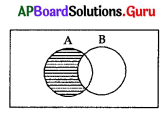A) A – B
B) A ∩ B
C) A Δ B
D) B – A
A) A – B

Question 143.
The shaded area in the figure is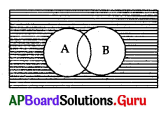A) A ∩ B
B) A’ ∩ B’
C) A’ ∪ B’
D) A ∪ B
B) A’ ∩ B’

Question 144.
The Venn Diagram by A ⊆ B is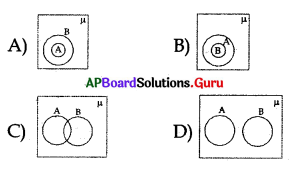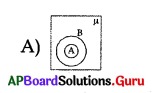Question 145.
The shaded area in the figure is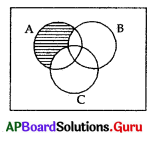A) A ∪ (B ∩ C)
B) A – (B ∩ C)
C) A ∩ (B ∪ C)
D) A – (B ∪ C)
D) A – (B ∪ C)

Question 146.
The “Venn Diagram “represents (A ∪ B) is .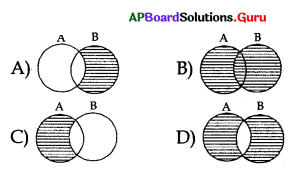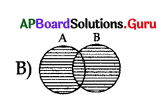Question 147.
Which of the following Venn Diagram represents (A – B) ?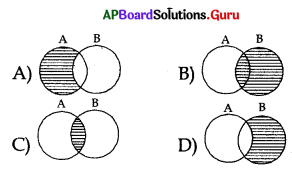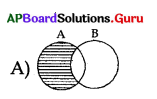Question 148.
The region representing A only is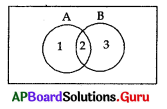A) 1, 2
B) 2, 3
C) 1
D) 3
C) 1

Question 149.
The region representing B – A is
A) 2, 3
B) 3
C) 1, 2
D) 1
B) 3

Question 150.
The region representing A Δ B is
A) 1, 3
B) 1
C) 3
D) 1, 2
A) 1, 3

Question 151.
The region representing (A ∪ B)’ is
A) 1, 2, 3
B) 2
C) 4
D) 1, 3, 4
C) 4

Question 152.
The region representing (A ∩ B)’ is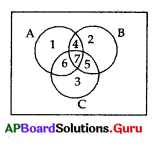A) 1, 2, 3
B) 4
C) 2
D) 1, 3, 4
D) 1, 3, 4Question 153.
In the above figure, the region represented by A is
A) 1, 2, 3
B) 1, 4, 6, 7
C) 2, 4, 5, 7
D) 1
B) 1, 4, 6, 7

Question 154.
In the above figure, the region represented by (A ∩ B) ∩ C is
A) 4, 5, 6, 7
B) 4, 5, 6
C) 7
D) 4, 6, 7